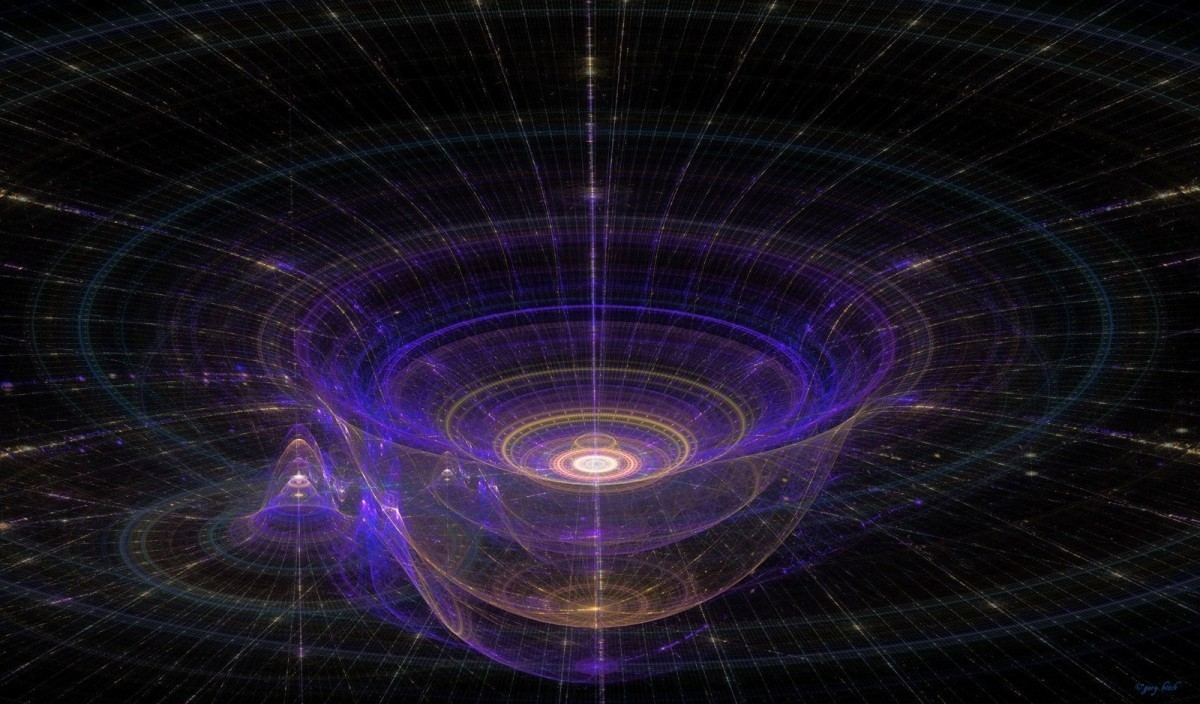# Is Gravity Quantized?

In Gravity by Brian Koberlein2 Comments

In physics there are two broad ways to look at the world. One is the classical realm of Newton and Einstein, where objects have definite form and interact in clearly determinate ways. The other is the quantum realm, where objects seem nebulous, with a strange mix of particle-like and wave-like behavior. The classical view gives us a wonderfully accurate description of everything from planets to baseballs. The quantum view is necessary to accurately describe the behavior of light and atoms. The classical world dominates on the scale of our daily lives, but nature seems to be rooted in quantum theory at its most basic level.

While both the classical and quantum approach are extremely accurate in their respective regimes, what happens in the intersection of the two regimes is still unclear. We don’t have a rigorous theory combining our classical and quantum models. We also don’t have certain key observational evidence, particularly in the nexus of quantum theory and gravity. But as quantum experiments increasingly study more massive objects and gravity experiments become increasingly sensitive, we’re approaching the point where “quantum gravity” experiments could be made. That’s the goal of a recently proposed experiment.

Since there isn’t yet a unified theory of quantum gravity, folks have instead focused on approximate approaches. One such approach is to add gravity to quantum theory a little bit at a time. This perturbative approach quantizes objects and their gravitational fields, and it works well for weak gravitational fields. One of the predictions of this approach is the existence of gravitons as the field quanta of gravity, much like photons are the field quanta of electromagnetism. However with stronger gravitational fields the approach becomes problematic. Basically, perturbative gravity builds upon itself in a way that is unphysical, so the model breaks down.

Another approach is known as the semi-classical method. Here gravity is treated as a field of space and time just as Einstein proposed, but the objects in spacetime are treated as quantum objects. The most famous prediction of this model is the Hawking radiation of black holes. The semi-classical model is not without its problems, particularly with strong gravitational fields, but for weak gravitational fields it reduces to the Schrödinger–Newton equation, which describes quantum objects interacting through classical Newtonian gravity.

What’s interesting is that these two approaches to gravity make fundamentally different predictions, even for weak gravitational fields. Either gravity is a classical background in which quantum objects interact, or both gravity and objects are quantized. This proposed experiment could determine which approach matches reality. Their idea is to take a charged disk of osmium with a mass of about a billionth of a gram and suspend it an electric field. This is small enough that its energy levels in the electric field would take on quantum behavior when cooled to temperatures a fraction of a Kelvin above absolute zero, but its also massive enough that its gravitational pull would affect the quantum behavior.

As the authors point out, the Schrödinger–Newton equation predicts energy levels that are different from perturbative approach. So such an experiment could determine whether semi-classical gravity is valid. It should be stressed that this is currently a proposal, not an actual experiment, but it could be a feasible way to bring gravity into the quantum realm.

Paper: André Großardt. Optomechanical test of the Schrödinger-Newton equation. arXiv:1510.01696 (2015).

This post originally appeared on Forbes.

1.